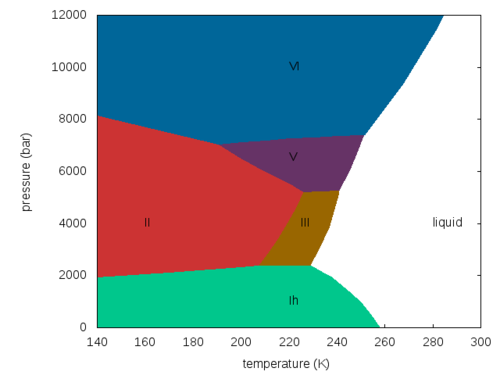2022: SklogWiki celebrates 15 years on-line

TIP4PQ/2005 model of water

(Redirected from TIP4PQ/2005)

The TIP4PQ/2005 model  is a re-parameterisation of the TIP4P/2005 potential for quantum path integral simulations of water, in particular for the ice phases. The difference between the TIP4P/2005 and the TIP4PQ/2005 models is the dipole moment, which increases from 2.305D to 2.38D thanks to the difference in the charges: TIP4P/2005 q(H) (e) is 0.5564 whereas for the TIP4PQ/2005 model this becomes 0.5764e.

Parameters$r_{\mathrm {OH}}$ (Å)$\angle$HOH , deg$\sigma$ (Å)$\epsilon/k$ (K) q(O) (e) q(H) (e) q(M) (e)$r_{\mathrm {OM}}$ (Å) 0.9572 104.52 3.1589 93.2 0 0.5764 -2q(H) 0.1546

Phase diagram

The phase diagram of the TIP4PQ/2005 model in the pressure-temperature plane (adapted from Fig. 3 of )Density maximum

The TIP4PQ/2005 model has a density maximum in the liquid phase at 280K .